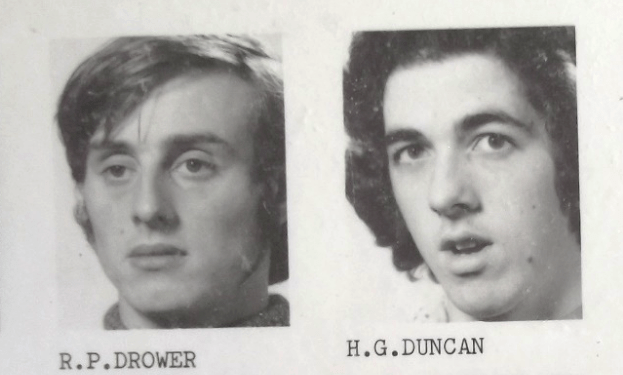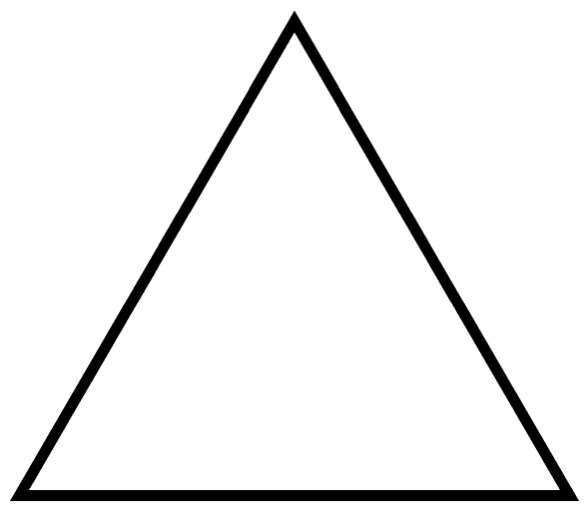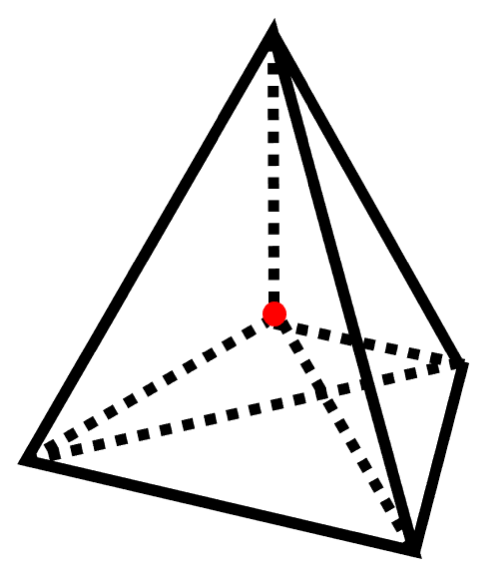# The first negative dimension

A tribute to alumni Roly Drower by Hugh DuncanForget 5D cinema and 3D glasses, as there might be something just as interesting to be seen below Ground Zero! It starts back in 1976, when the world was young and still in black and white. I met Roland Drower at UCL when we both started our first year of an astronomy degree. Roland introduced me to the idea that there might be a ‘minus one’ dimension! I don’t know if he had actually come up with this idea himself or if it had been shown to him, but he had thought up several novel ideas so this one is quite likely his. The date was Tuesday 23rd November 1976 and my diary comment was: Roly and I got talking about minus one dimensions. He showed me the Pascal’s Triangle formula applied to simple shapes. I was impressed.

## Symmetry with Pascal’s Triangle

Roland’s reasoning went something like this: consider the simplest ‘straight sided’ or Euclidean shape for each dimensional space chosen. The simplest zero-dimensional shape is a point. Such a shape can be characterised by being made of just one zero-dimensional facet—ie a point. The simplest one-dimensional shape is a line, made of one edge joined between two points. It is composed of two zero-dimensional facets—the two points or vertices (one at each end)—plus just one one-dimensional facet: the line between them, the edge. The simplest in two dimensions is a triangle, in three dimensions a tetrahedron and in four a pentatope. The characteristics of each of these simple shapes are shown in the table below:

Dimension of object Name of shape Shape No. of vertices (0D) No. of edges (1D) No. of areas (2D) No. of volumes (3D) No. of hypervolumes ((>3)D)
0 Point . 1
1 Line _____ 2 1
2 Triangle3 3 1
3 Tetrahedron4 6 4 1
4 Pentatope5 10 10 5 1

Each shape in this simple series is created by adding one more vertex in the next additional dimension and connecting it to the previously existing points by edges, which in turn create faces, volumes and so on. It is not surprising therefore that there is a pattern in the numbers of each dimensional facet. In fact, the table displays the well-known Pascal’s Triangle of numbers, except missing the left hand column of ‘ones’.

While there is already a derivation for this pattern of numbers, it is curious that this table is so close to being Pascal’s Triangle. It is almost as if the table itself is dropping hints that there is a missing left hand column of ones! There is often much symmetry in the world, such as wave-particle duality, equal numbers of quarks and leptons, the patterns in The Periodic Table, and so on. Thus, if there is complete symmetry in these simple shapes from each dimension, then let us assume that the almost complete Pascal’s Triangle that we see is in fact a complete triangle of numbers. Let us call it the first conjecture:

The dimensional facets of the simplest shape in each dimension follows Pascal’s triangle.

There, we did it! See below the now modified table including that left hand column of ‘ones’ added and look what dimension it must have: negative one! It also brings into existence a dimension below zero, if the pattern were to continue!

Shape Minus one (-1D) Vertices (0D) Edges (1D) Areas (2D) Volumes (3D) Hypervolumes ((>3)D)
Simplest 1
Point 1 1
Line 1 2 1
Triangle 1 3 3 1
Tetrahedron 1 4 6 4 1
Pentatope 1 5 10 10 5 1

According to this symmetry theory, all of the simplest objects contain one facet of the first negative dimension. Even a point, that was previously completely described as being made of one 0-dimensional facet, is now seen to also contain one example of the new $-1$-dimensional facet. What is also interesting is that in order to complete the table, we have now created an object even more ‘simple’ than a point—the minus one itself, which is made up of only one $-1$-dimensional facet and nothing else. Not even a point. Note that this table does not include diagrams of these shapes. What would a $-1$D object even look like?! Also note that the sum of the odd dimensional facets is equal to the sum of the even dimensional facets.

## The dimension of infinity

The second idea that Roland showed me was to determine the dimension of what is infinitely far away from a given shape being considered, taking into account the dimension of the space in which the shape sits. For example: take a point (0 dimensions) sitting on a line (one dimension). We ask: what is the dimension of the object that is infinitely far from this point? Let’s just call this thing ‘infinity’ for now. Two points can be placed equidistant on either side of our point (see below). They are not infinitely far away, but they can be moved as far away as required—to infinity and beyond! So, the infinity for a point in a one-dimensional space is zero-dimensional: two points, one at each of the far ends of this 1D space.

Now take a point (0 dimensions), in two-dimensional space (a plane). Another point can be chosen at some distance from our point. And there are other points at the same distance: a ring of them. This ring of points can be expanded to become infinitely far away (see below). Thus for a zero-dimensional shape (point) in a two-dimensional space (plane), its infinity is one-dimensional (a circular line).

Consider also a line (1 dimension) in our 2D world (see above). We can select equidistant points on each side of the line. They are not the same distance from every point on our line—they are obviously closer to the points in the middle. Now gradually move these two points further away and they eventually become equidistant from all points on the line as they approach infinite distance. So, for a one-dimensional shape (line) in two-dimensional space (plane), infinity has zero dimensions (two points).

Now moving on to three dimensions, consider a point (0 dimensions) sitting in this 3D space (see below). Equidistant points can be found in all directions from our point. In other words, a spherical surface is equidistant from our point, and if moved as far away as possible, our zero-dimensional object in three-dimensional space has an infinity that has two dimensions (a spherical surface).

Staying in three dimensions, now consider a line (one dimension). There is a ring of points that are equidistant from the closest point of the line (see above). Expand this ring of points to as far away as possible, and this ring will be equidistant from all the points on the line. Thus, for a one-dimensional shape (line) in three-dimensional space, infinity has one dimension (a circular line).

Finally consider a plane (2 dimensions) in three-dimensional space (see below). Consider two points equidistant from the plane: one above the plane and one below, that are at the same distance from their closest point on the plane. Move these two points off to infinity in both directions and eventually, they will be equidistant from all points on the plane. So, for a two-dimensional shape (plane) in three-dimensional space, infinity has zero dimensions (two points).

All these results are summarised in the table below:

Dimension of space Dimension of object Dimension of infinity
0 0 ? (-1)
1 0 0
1 1 ? (-1)
2 0 1
2 1 0
2 2 ? (-1)
3 0 2
3 1 1
3 2 0
3 3 ? (-1)

There is a pattern. As the dimension of the object goes up, the dimension of infinity goes down. The lines in the table with the question marks for infinity seem to suggest that for an object with the same dimension as its space, infinity will have a dimension of $-1$! In fact, there is a simple equation, the Infinity Dimension Equation:
$\textrm{dimension of space }(S) – 1 = \textrm{dimension of shape }(H) + \textrm{dimension of infinity } (Y)$
$S-1=H+Y$
So for example, for a cube sitting in a three-dimensional space, its infinity has -1 dimensions. The same for a square sitting in a plane, a line on a line and a point in a point (weird!).

## Coxeter’s Indirect Reference to -1D

On page 165 (Ch.IX) of Coxeter’s excellent book on Regular Polytopes, he refers to Euler’s formula as generalised by Schlafli. For a line (1D), polygon (2D), to polytope or ‘shape’ of higher dimension $n$, the number $N$ of odd-dimensional elements minus the number of even-dimensional elements is zero. In other words:
$N_{-1}-N_0+N_1-N_2+N_3-N_4 \dots \pm N_{n-1} \mp N_n = 0$
which is exactly what has been said above! Not only that, the term $N_{-1}$ is the number of minus one-dimensional facets. I count that as supporting evidence! On behalf of Roland, I feel he is vindicated.

## Conclusion

There is a lot of conjecture above, I realise this, but doesn’t it feel like the patterns are advertising these negative dimensions? I did try to track down Roland Drower for more answers but sadly, he passed away in 2008. I did find his sister Jill (who was also at UCL) who said she didn’t even know of his mathematical dabblings! She still has his old papers, so the story may not be over yet. For those who would like to find out more about this unique UCL alumni, you can do so here.Hugh Duncan graduated from UCL having studied astronomy. He taught physics and maths at the International School of Nice for over thirty years. Hugh was a punk when he started UCL in 1976 and guess what, he still is! Check out his band Old Age Spies on YouTube. He recently retired and has just had his first science fantasy book published ‘Life On Mars – The Vikings Are Coming’ inspired by his time at UCL. Check it out too!